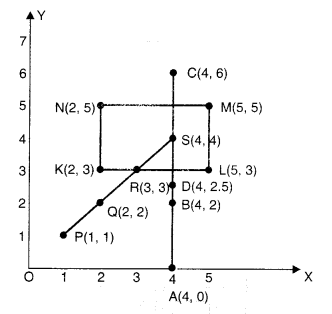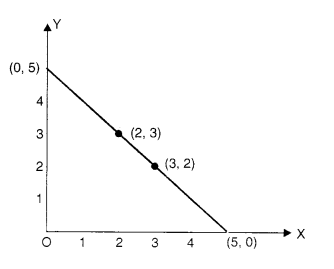NCERT Solutions for Class 8 Maths Chapter 15 Introduction to Graphs Ex 15.2 are part of NCERT Solutions for Class 8 Maths. Here we have given NCERT Solutions for Class 8 Maths Chapter 15 Introduction to Graphs Ex 15.2.

 Board CBSE Textbook NCERT Class Class 8 Subject Maths Chapter Chapter 15 Chapter Name Introduction to Graphs Exercise Ex 15.2 Number of Questions Solved 4 Category NCERT Solutions

## NCERT Solutions for Class 8 Maths Chapter 15 Introduction to Graphs Ex 15.2

Question 1.
Plot the following points on a graph sheet. Verify if they lie on a line.
(a) A(4, 0), B(4, 2), C(4, 6), D(4, 2.5)
(b) P(l, 1), Q(2, 2), R(3, 3), S(4, 4)
(c) K(2, 3), L(5, 3), M(5, 5), N(2, 5)
Solution.(a) The points lie on a line.
(b) The points lie on a line.
(c) The points do not lie on a line.

Question 2.
Draw the line passing through (2, 3) and (3, 2). Find the coordinates of the points at which this line meets the x-axis and y-axis.
Solution.
The coordinates of the points at which this line meets the x-axis and y-axis are (5, 0) and (0, 5) respectively. See the graph given above.Question 3.
Write the coordinates of the vertices of each of these adjoining figures.Solution.
O → (0, 0)
A → (2,0)
B → (2, 3)
C → (0,3)

P → (4, 3)
Q → (6, 1)
R → (6, 5)
S → (4, 7)

K → (10, 5)
L → (7, 7)
M → (10, 8)

Question 4.
State whether True or False. Correct that is false.
(i) A point whose x-coordinate is zero and y-coordinate is non-zero will lie on the y-axis.
(ii) A point whose y-coordinate is zero ‘ and x-coordinate is 5 will lie on y-axis.
(iii) The coordinates of the origin are (0, 0).
Solution.
(i) True
(ii) False; A point whose y-coordinate is zero and x-coordinate is 5 will lie on x-axis.
(iii) True

We hope the NCERT Solutions for Class 8 Maths Chapter 15 Introduction to Graphs Ex 15.2 help you. If you have any query regarding NCERT Solutions for Class 8 Maths Chapter 15 Introduction to Graphs Ex 15.2, drop a comment below and we will get back to you at the earliest.